# Etale cohomology of simplicial schemes

• ## General Introduction

See stuff on simplicial schemes in my private notes. See also Motivic homotopy theory.

<]]>

<]]>
• ## Online References

Article by Quick?

<]]>
• ## Paper References

Friedlander: Etale homotopy of simplicial schemes

Deligne: Hodge III

<]]>
• ## Definition

Def. Etale site of a simplicial scheme. Let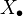be a simplicial scheme. An object in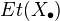is an etale map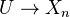for some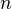. Maps are commutative squares of the obvious form, where the bottom map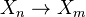is a specified structure map of. A covering ofis a family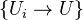of etale morphisms of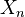-schemes such that the images of the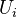cover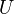.

(Many more interesting details in Friedlander)

A sheaf is a contravariant functor on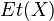satisfying the sheaf condition. This is equivalent to a collection of sheaves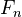onsatisfying some compatibility criteria.

Now etale cohomology is defined in the usual way, as the right derived functors of the "global sections functor". The "global sections functor" here is the map sending a sheaf of abelian groups to the kernel of the map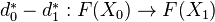. Equivalently,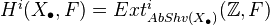Can also define etale cohomology of bisimplicial schemes.

<]]>

<]]>

<]]>

<]]>

<]]>
• ## Computations and Examples

Can use hypercoverings/Cech cohomology. See Friedlander.

<]]>

<]]>

<]]>

<]]>